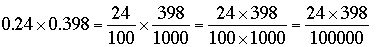SEARCH HOMEMath Central Quandaries & QueriesQuestion from telly, a student: how to do this sum 0.24*0.398We have two responses for you

Telly,

Think of 0.24 as 24/100 and 0.398 as 398/1000 thenThus you can multiply 0.24 and 0.398 my multiplying 24 and 398 and then dividing by 100,000. Thus calculate 24 × 398 and then move the decimal place 5 places to the left.

Penny

Telly,

Use exactly the same method you would use to find

24 * 398

but adjust the place value. Three ways to do this:

1. Work out the place value for each partial product. Thus

0.24
0.398
----------
[.008*0.24 =] 0.00192
(etc)
Your teacher may prefer this method if you have to show your work in detail

2. You can actually find 24 * 398 and fix place value after the calculation is completed. To get from 0.24 to 24 you have to shift the place value 2 places. To get from 0.398 to 398 you have to shift it 3 places. So to get the final answer you have to shift back 5 places.